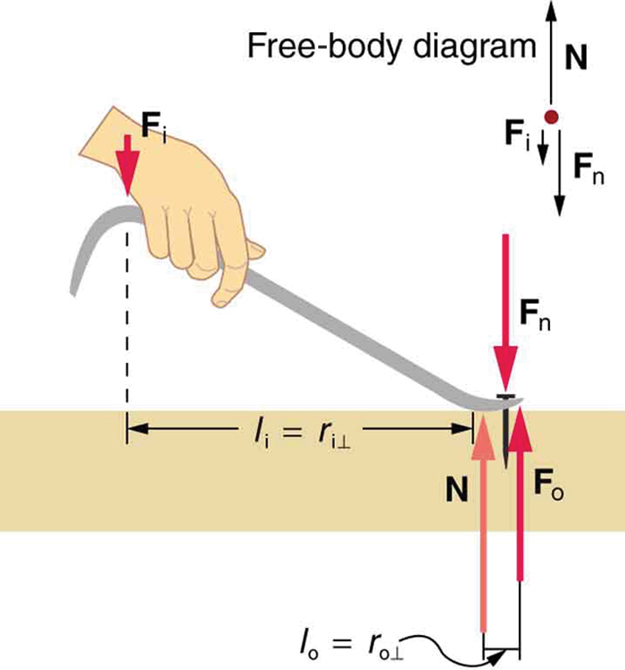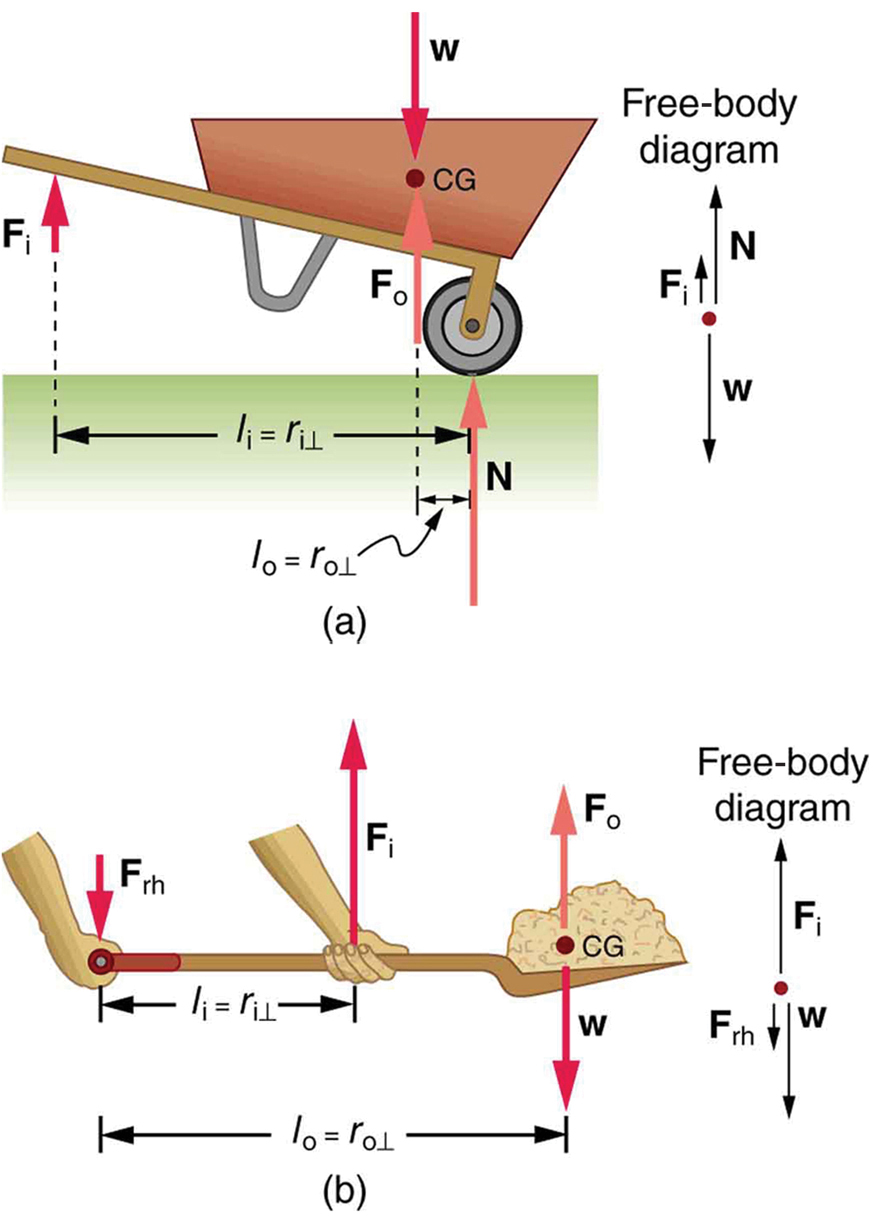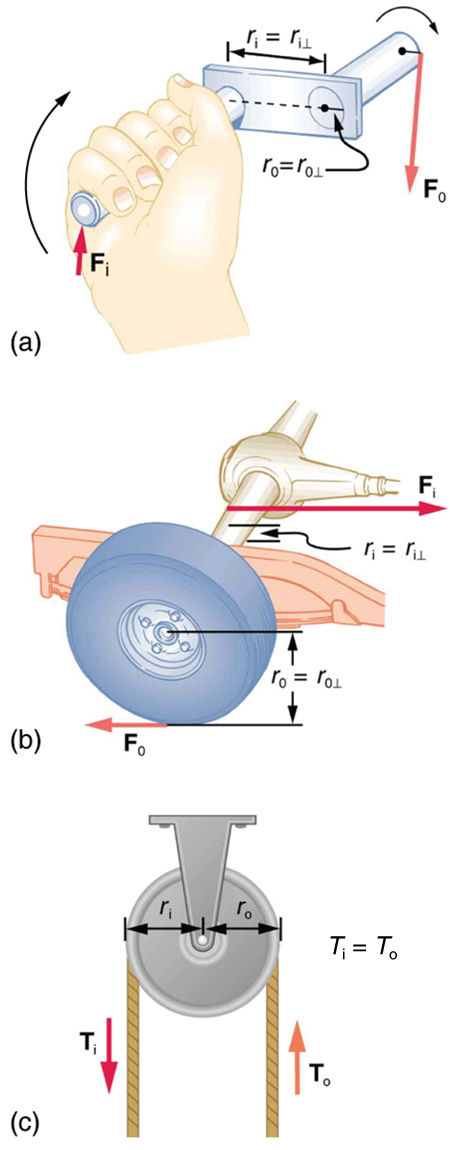### Summary

Simple machines are devices that can be used to multiply or augment a force that we apply – often at the expense of a distance through which we apply the force. The human body can be compared to simple machines depending on muscle attachment and weight of the limb. The word for “machine” comes from the Greek word meaning “to help make things easier.” Levers, gears, pulleys, wedges, and screws are some examples of machines. The ratio of output to input force magnitudes for any simple machine is called its mechanical advantage (MA).

MA = (F output) / ( F input )

One of the simplest machines is the lever, which is a rigid bar pivoted at a fixed place called the fulcrum. Torques are involved in levers, since there is rotation about a pivot point. Distances from the physical pivot of the lever are crucial, and we can obtain a useful expression for the MA in terms of these distances.Figure 1. A nail puller is a lever with a large mechanical advantage. The external forces on the nail puller are represented by solid arrows. The force that the nail puller applies to the nail (Fo) is not a force on the nail puller. The reaction force the nail exerts back on the puller (Fn) is an external force and is equal and opposite to Fo. The perpendicular lever arms of the input and output forces are li and l0.

Figure 1 shows a lever type that is used as a nail puller. Crowbars, seesaws, and other such levers are all analogous to this one. $\boldsymbol{\vec{\textbf{F}}_{\textbf{i}}}$ is the input force and $\boldsymbol{\vec{\textbf{F}}_{\textbf{o}}}$ is the output force. There are three vertical forces acting on the nail puller (the system of interest) – these are $\boldsymbol{\vec{\textbf{F}}_{\textbf{i}},\: \vec{\textbf{F}}_{\textbf{o}}}$ and $\vec{\textbf{N}}.$ $\boldsymbol{\vec{\textbf{F}}_{\textbf{n}}}$ is the reaction force back on the system, equal and opposite to $\boldsymbol{\vec{\textbf{F}}_{\textbf{o}}}.$ (Note that $\boldsymbol{\vec{\textbf{F}}_{\textbf{o}}}$ is not a force on the system.) $\vec{\textbf{N}}$ is the normal force upon the lever, and its torque is zero since it is exerted at the pivot. The torques due to $\boldsymbol{\vec{\textbf{F}}_{\textbf{i}}}$ and $\boldsymbol{\vec{\textbf{F}}_{\textbf{n}}}$ must be equal to each other if the nail is not moving, to satisfy the second condition for equilibrium (net τ = 0). (In order for the nail to actually move, the torque due to $\boldsymbol{\vec{\textbf{F}}_{\textbf{i}}}$ must be ever-so-slightly greater than torque due to $\boldsymbol{\vec{\textbf{F}}_{\textbf{n}}}$.) Hence,

$\boldsymbol{l_{\textbf{i}}F_{\textbf{i}}=l_{\textbf{o}}F_{\textbf{o}}}$

where li and lo are the distances from where the input and output forces are applied to the pivot, as shown in the figure. Rearranging the last equation gives

$\boldsymbol{\frac{F_{\textbf{o}}}{F_{\textbf{i}}}}\boldsymbol{=}\boldsymbol{\frac{l_{\textbf{i}}}{l_{\textbf{o}}}}.$

What interests us most here is that the magnitude of the force exerted by the nail puller, Fo, is much greater than the magnitude of the input force applied to the puller at the other end, Fi. For the nail puller,

$\boldsymbol{\textbf{MA}\:=}\boldsymbol{\frac{F_{\textbf{o}}}{F_{\textbf{i}}}}\boldsymbol{=}\boldsymbol{\frac{l_{\textbf{i}}}{l_{\textbf{o}}}}.$

This equation is true for levers in general. For the nail puller, the MA is certainly greater than one. The longer the handle on the nail puller, the greater the force you can exert with it.

Two other types of levers that differ slightly from the nail puller are a wheelbarrow and a shovel, shown in Figure 2. All these lever types are similar in that only three forces are involved – the input force, the output force, and the force on the pivot – and thus their MAs are given by $\boldsymbol{\textbf{MA}=\frac{F_{\textbf{o}}}{F_{\textbf{i}}}}$ and $\boldsymbol{\textbf{MA}=\frac{d_1}{d_2}},$ with distances being measured relative to the physical pivot. The wheelbarrow and shovel differ from the nail puller because both the input and output forces are on the same side of the pivot.

In the case of the wheelbarrow, the output force or load is between the pivot (the wheel’s axle) and the input or applied force. In the case of the shovel, the input force is between the pivot (at the end of the handle) and the load, but the input lever arm is shorter than the output lever arm. In this case, the MA is less than one.Figure 2. (a) In the case of the wheelbarrow, the output force or load is between the pivot and the input force. The pivot is the wheel’s axle. Here, the output force is greater than the input force. Thus, a wheelbarrow enables you to lift much heavier loads than you could with your body alone. (b) In the case of the shovel, the input force is between the pivot and the load, but the input lever arm is shorter than the output lever arm. The pivot is at the handle held by the right hand. Here, the output force (supporting the shovel’s load) is less than the input force (from the hand nearest the load), because the input is exerted closer to the pivot than is the output.

### Example 1: What is the Advantage for the Wheelbarrow?

In the wheelbarrow of Figure 2, the load has a perpendicular lever arm of 7.50 cm, while the hands have a perpendicular lever arm of 1.02 m. (a) What upward force must you exert to support the wheelbarrow and its load if their combined mass is 45.0 kg? (b) What force does the wheelbarrow exert on the ground?

Strategy

Here, we use the concept of mechanical advantage.

Solution

(a) In this case, $\boldsymbol{\frac{F_{\textbf{o}}}{F_{\textbf{i}}}=\frac{l_{\textbf{i}}}{l_{\textbf{o}}}}$ becomes

$\boldsymbol{F_{\textbf{i}}=F_{\textbf{o}}}\boldsymbol{\frac{l_{\textbf{o}}}{l_{\textbf{i}}}}.$

Adding values into this equation yields

$\boldsymbol{F_{\textbf{i}}=(45.0\textbf{ kg})(9.80\textbf{ m/s}^2)}\boldsymbol{\frac{0.075\textbf{ m}}{1.02\textbf{ m}}}\boldsymbol{=\:32.4\textbf{ N}}.$

The free-body diagram (see Figure 2) gives the following normal force: Fi + N = W. Therefore, N = (45.0 kg)(9.80 m/s2) -32.4 N = 409 N. N is the normal force acting on the wheel; by Newton’s third law, the force the wheel exerts on the ground is 409 N.

Discussion

An even longer handle would reduce the force needed to lift the load. The MA here is MA=1.02/0.0750=13.6.

Another very simple machine is the inclined plane. Pushing a cart up a plane is easier than lifting the same cart straight up to the top using a ladder, because the applied force is less. However, the work done in both cases (assuming the work done by friction is negligible) is the same.

A crank is a lever that can be rotated 360° about its pivot, as shown in Figure 3. Such a machine may not look like a lever, but the physics of its actions remain the same. The MA for a crank is simply the ratio of the radii ri/r0. Wheels and gears have this simple expression for their MAs too. The MA can be greater than 1, as it is for the crank, or less than 1, as it is for the simplified car axle driving the wheels, as shown. If the axle’s radius is 2.0 cm and the wheel’s radius is 24.0 cm, then MA=2.0/24.0=0.083 and the axle would have to exert a force of 12,000 N on the wheel to enable it to exert a force of 1000 N on the ground.Figure 3. (a) A crank is a type of lever that can be rotated 360º about its pivot. Cranks are usually designed to have a large MA. (b) A simplified automobile axle drives a wheel, which has a much larger diameter than the axle. The MA is less than 1. (c) An ordinary pulley is used to lift a heavy load. The pulley changes the direction of the force T exerted by the cord without changing its magnitude. Hence, this machine has an MA of 1.

# Section Summary

• Simple machines are devices that can be used to multiply or augment a force that we apply – often at the expense of a distance through which we have to apply the force.
• The ratio of output to input forces for any simple machine is called its mechanical advantage
• A few simple machines are the lever, nail puller, wheelbarrow, crank, etc.

### Conceptual Questions

1: Why are the forces exerted on the outside world by the limbs of our bodies usually much smaller than the forces exerted by muscles inside the body?

2: Explain why the forces in our joints are several times larger than the forces we exert on the outside world with our limbs. Can these forces be even greater than muscle forces (see previous Question)?

## Glossary